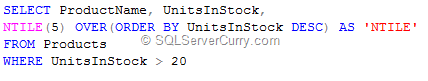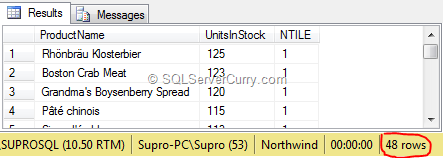### Group Results and Rank Them in SQL Server using NTILE

The SQL Server NTILE() function divides the result set into a specified number of even sized group (approximate division) and assigns a ranking value to these groups.

The NTILE() function is similar to other ranking functions like the RANK() and DENSE_RANK(), except that NTILE takes one parameter of type int/bigint that specifies the number of groups into which each partition must be divided.

Let us see an example. We will query the Products table of the Northwind database and divide it into 5 groups ordered by the UnitsInStock:OUTPUTOn running the query, the results are divided into 5 groups, but because the total number of rows (48) is not divisible by the number of groups (5), NTILE puts 10 rows in the first three group and the remaining two groups have 9 rows each.